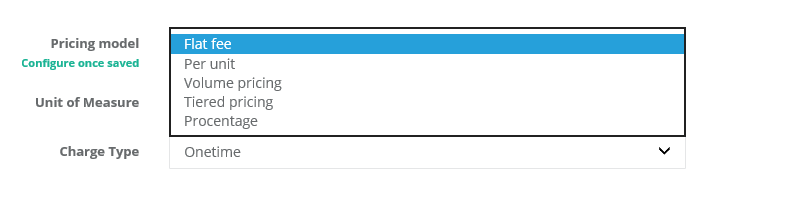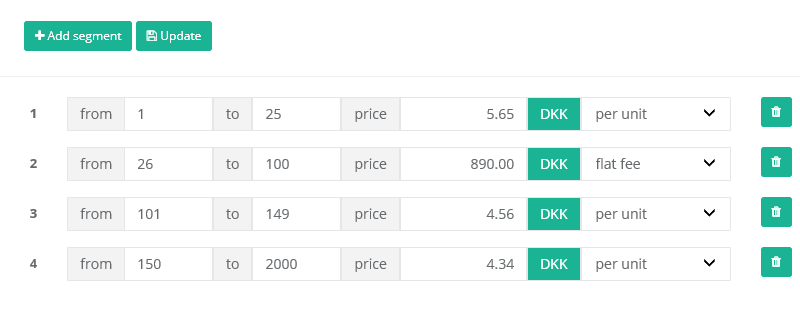Upodi provides support for various monetization models. A monetization model is componentized by a pricing model and a charge type.

Upodi support

• ﻿[Flat fee](🔗﻿)﻿

• ﻿[Per unit](🔗﻿)﻿

• ﻿[Tiered pricing](🔗﻿)﻿

• ﻿[Volume pricing](🔗﻿)﻿

• ﻿[Procentage total](🔗﻿)﻿

The following outlines the different use.﻿

Due to support of underlying finance systems (ERP), Upodi will handle all decimals when calculating tier and volume pricing, by supplementing quantity with 1 x the calculated price and adding the full quantity of this to the invoice line. ERP systems often calculate pricing of amount x quantity price, rendering the full amount wrong if decimals becomes larger than 2.

### ### **Flat-fee**

A _flat fee_ pricing model bills a subscription a fixed amount in the term of the subscription. Flat fee pricing models ignore the quantity of the subscription charge, however, a subscription charge quantity of 0 will ignore the fee in a billing cycle.

Flat fee pricing models are usually used for sign-up fees, cancellation fees and based level subscription fees.

### ### **Per-unit**

A per-unit pricing model, multiplies the per-unit amount with the quantity of a subscription charge. The following formular is used

﻿

Per unit fees are often used in SaaS subscription plans (per user) or mobile data plans (per gb data).

### ### **Tiered pricing**

A tiered pricing model offers a flexible billing based on the quantity amount of a subscription. A tiered pricing model is calculated based on the quantity tier and type of that tier multiplying each tier of the full quantity.﻿

Each tier can be charged by _per-unit pricing_ level (see above), or a _[Flat fee](🔗﻿)_ level (see above).

#### #### Example:

A tiered pricing model is calculated by the sum of all tiers matching the volume. Let's try to define this by looking at a sample table.

 Tier Pricing level Pricing Range A Per-unit \$20 per unit 1-100 B Per-unit \$15 per unit 101-200 C Per-unit \$10 per unit 201-300

A tiered pricing model for a quantity of 130, will sample calculation of _tier A_ plus _tier B_ but not _tier C_. Calculation is done as follows:

﻿

### ### **Volume pricing**

A volume pricing is often called a "discount ladder". A volume pricing models looks for a given tier of the volume pricing model, and calculates the total quantity of this tier.

#### #### Example

Using the sample table from above (tiered pricing), a volume pricing model, calculated with a quantity of 130, is done as follows. 130 matches tier B:

﻿

### ### **Procentage total**

Upodi only support one _Procentage total_ pricing model per product plan. In addition, the _Procentage total_ pricing model, is the last pricing model to be calculated on if present.

The calculation of this pricing model, is done as a procentage of total, of all previous charges summed together. Hence the name.

﻿

The _Procentage total_ pricing model, can be used to apply credit card (i.e. 0.5%) feeds or typed discounts onto a product plan.

## ## Questions?

We're always happy to help with code or other questions you might have! **Search** our documentation or contact **helpdesk**. You can also chat live with us using the Intercom icon.